# Reduced order models for bifurcation problems in nonlinear PDEs

A beam under compression, the flow in heart’s chambers and the evolution of a particle’s wave-function. We are surrounded by these phenomena in our daily life, and a strategic task for the progress is to develop a deep understanding of their complex behaviour.

The above physical processes can be modelled by the so called Partial Differential Equations (PDEs), that describe through differential operators the relations between the temporal/spatial unknowns of the problem under investigation.

Thinking about how differently a rubber object responds to compression with respect to a steel one, it is clear that the analysis of the models has to carry out some information about their physical properties. This is generally achieved by employing a parameter dependence of the PDEs.

Solving analytically such complex equations is in most cases impossible, thus advanced numerical methodologies can be implemented to approximate their solutions. However, despite the increasing computational resources, accurate enough numerical simulations are usually too much time-demanding, such that a complete analysis of their behaviour with respect to the parameter considered becomes impracticable.

The aforementioned physical phenomena are characterised by their complexity, which reflects into nonlinear relations that worsen the investigation. Indeed, such non-linearities usually originate the so called bifurcating phenomena, i.e. sudden changes in the behaviour of the solutions, and could represent a critical source of issues for their numerical approximation. In fact, while from the physical point of view this translates into the non-uniqueness of the state for a given parameter, on the other hand the mathematical model becomes singular in such critical points where the bifurcation occurs.

We focused on the analysis and the development of efficient Reduced Order Models (ROMs) for the investigation of complex bifurcating phenomena held by non- linear parametrised PDEs in many physical contexts, from Continuum Mechanics to Quantum Mechanics passing through Fluid Dynamics.

## Methodology

The numerical investigation of complex physical behaviour, which entails bifurcating phenomena, usually required fine discretisation of the domain in which the problem is posed. The presence of nonlinearities and the parameter dependence lead to a multi-query context that could represent a bottleneck for a suitable study. In fact, when dealing with bifurcations, one needs to compute the numerical approximation of the solution for many instances of the parameter in order to discover the critical points of the model and its post-bifurcating behaviour.

Moreover, the analysis becomes even more complicated if the goal is a complete reconstruction of all the possible solutions that the model could admit. Finally, a key aspect in these contexts is the stability analysis of the discovered solutions, which can be studied by means of specific eigenvalue problems.

The numerical approximation of such models requires a combination of different methodologies. To obtain an high fidelity approximation of the problem, we considered as Full Order Model (FOM) the Finite Element method, which we combined with a continuation method, to follow the solution varying the parameter, and to the Newton method which serves to linearise the equation.

We tackled the huge computational cost that such investigation would require by means of the ROMs. In particular, we developed a branch-wise algorithm based on the POD technique which aims at efficiently reconstructing the bifurcating behaviour projecting the governing equations into a low dimensional manifold spanned by global basis functions.

The key ingredient to recover the efficiency and allow for a complete real-time investigation of the parameter space in a multi-query context is the offline-online paradigm. Indeed, one assumes to decouple the computation such that the high fidelity quantities can be precomputed offline during an expansive phase, while the actual investigation of the parameter dependence is studied online, assembling reduced quantities with cheaper costs.

Unfortunately, the nonlinear terms appearing in the models we considered, compromise this decoupling. For this reason, affine-recovery techniques as the Empirical Interpolation Method (EIM) are fundamental to obtain rapid evaluations during the online phase. Despite this, sometimes they can add a further impracticable cost, even for the offline stage: different methodologies have to be considered.

## Applications

A wide variety of models have been studied with the twofold motivation of investigating the physics of their bifurcation phenomena and show the applicability of the ROMs strategies we have developed in the non-uniqueness context.

An example of a sudden change of the state solution with respect to a (compression) parameter is the buckling of plates, which is modelled through the Von Kármán equations .
Remaining in the context of deformation of solids, one can also study the buckling of beams, which is governed by a class of models called Hyperelastic equations .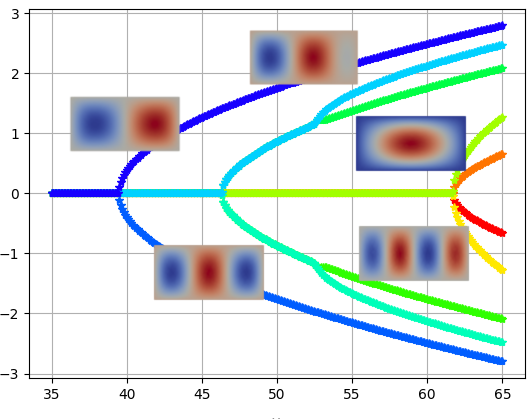Reduced bifurcation diagram of the Von Kármán equation for a rectangular two-dimensional plate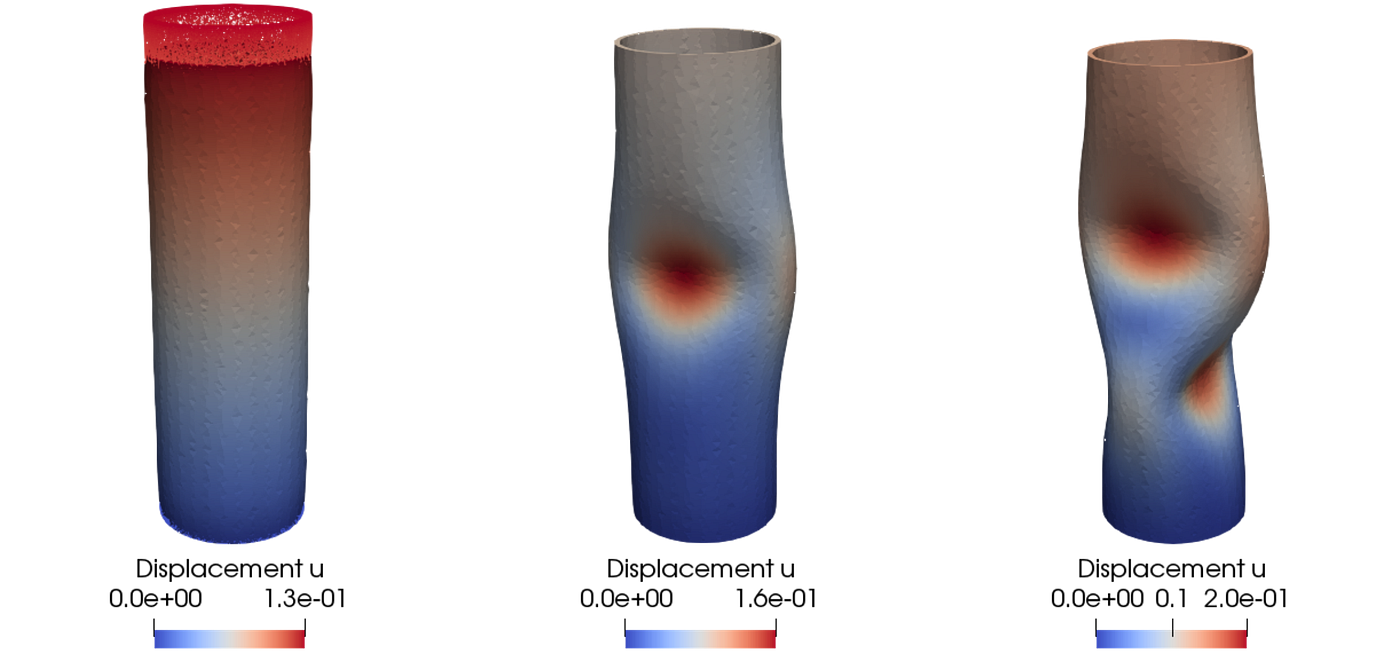Different buckling modes for a three-dimensional beam with annulus section

A different perspective is given by the analysis of the density of particle’s wave-function in a Bose-Einstein condensate . In fact, while varying the chemical potential many different configurations can coexist due to the complex nonlinear Schrödinger equation which describes their behaviour.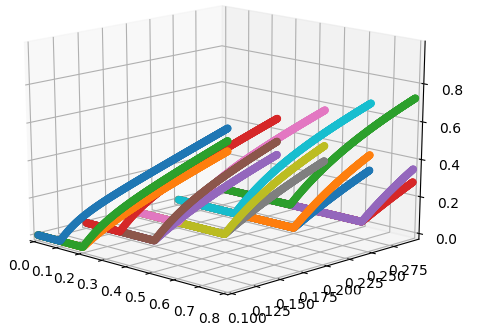Reduced basis bifurcation diagram for the Schrödinger equation with multiple parameter (trapping strength)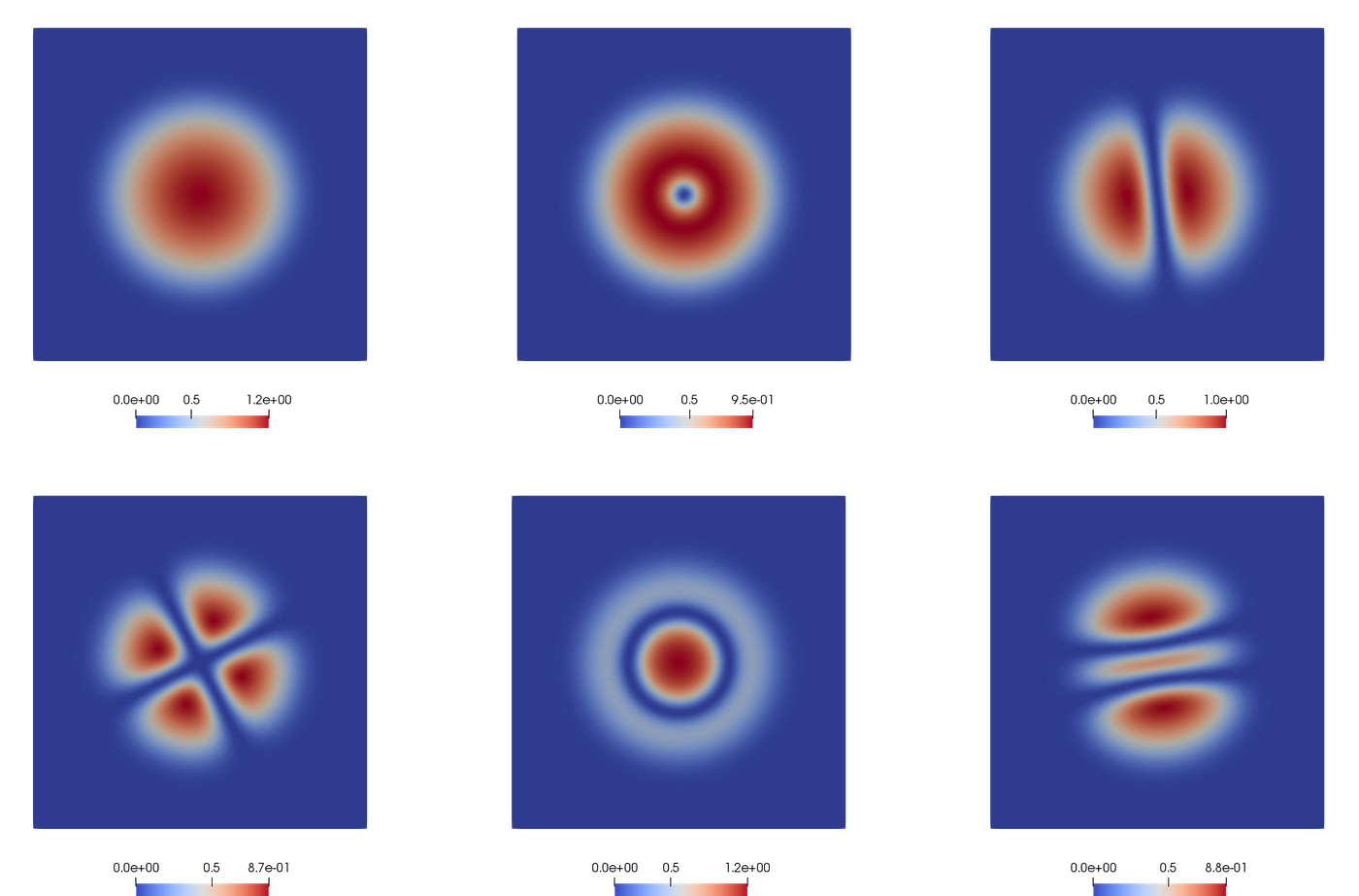Coexisting states for the density function of a single-particle wave-function

Finally, interesting bifurcating phenomena can be discovered in fluid dynamics applications governed by the Navier-Stokes equations .
Here we consider a model of a cardiac disease, called mitral valve regurgitation. This phenomenon can be clinically detected through echocardiography, it is called the Coanda effect, and expresses the tendency of a fluid jet to be attracted to a nearby surface. This represents an issue from the medical point of view, because a wall-hugging jet might lead to inaccurate echocardiography measurements.

A fluid, characterised by an high viscosity, presents a jet which is symmetric w.r.t. the horizontal axis. Furthermore, a pair of vortices, called Moffatt eddies, form downstream of the expansion. Lowering the viscosity, the inertial effects of fluid become more important and the two symmetric recirculation regions break the symmetry. Indeed, as the length of the recirculation zones increases, one can observe a non-uniform decrease of the pressure along the vertical axis. Thus, when we reach the aforementioned critical value, one recirculation zone expands whereas the other shrinks, giving rise to an asymmetric jet, which represents the wall hugging behaviour.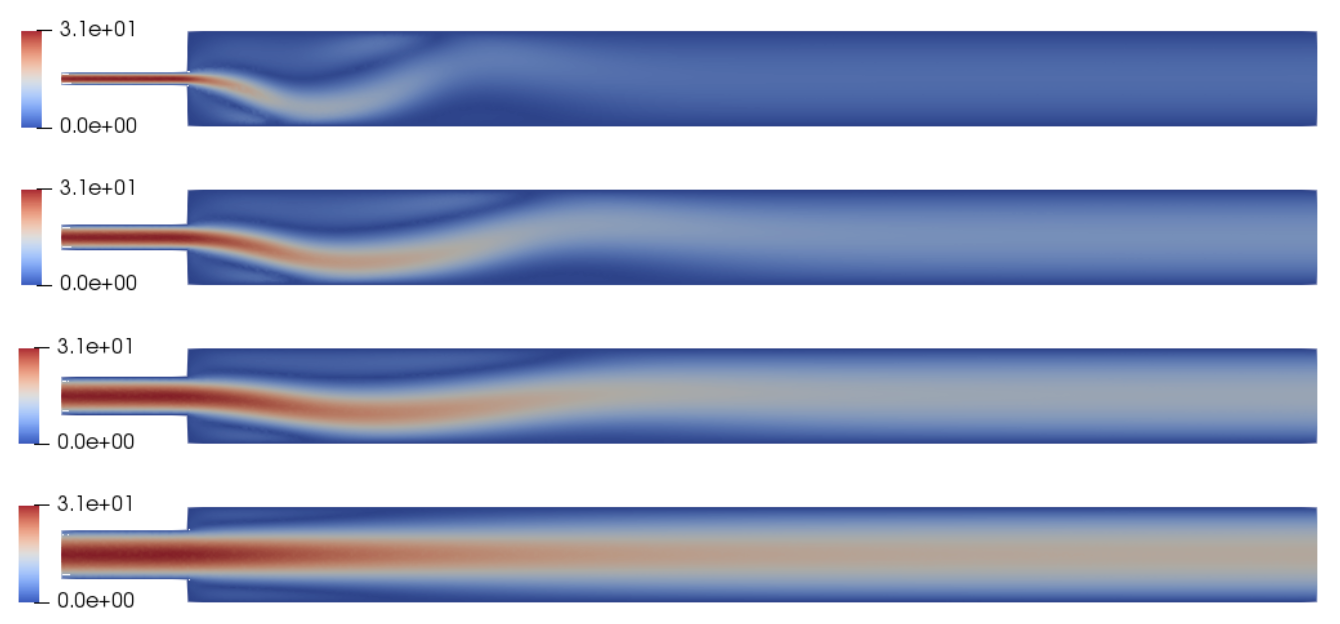Reduced basis bifurcation diagram for the Navier-Stokes equations in the multi-parameter case (inlet width)

## Further investigations

Of course, many issues and questions arise during the analysis of these complex models.

1. Which approaches can we consider as alternatives to the affine-recovery techniques?
Novel machine learning methodologies can be adapted to the ROMs paradigm by means of Artificial Neural Networks (ANNs), constituting a non-intrusive way to re- cover the online efficiency while discovering manifold’s characteristic features .
2. It is possible to develop an automatic methodology that guides our algorithm towards all the coexisting solutions for a given instance of the parameter?
To answer this question we analysed and developed a reduced deflation method which prevents the system to converge to already known solutions, enabling the discovery of new ones .
3. Can we somehow act on the system to steer the bifurcating solutions towards states with desired properties?
We deeply investigated how Optimal Control Problems (OCPs) can be designed to this goal, while studying their stability properties .

The numerical results presented have been performed with RBniCS library , developed at SISSA mathLab, which is an implementation in FEniCS of several reduced order modelling techniques; we acknowledge developers and contributors to both libraries.

## References

 F. Pichi and G. Rozza. Reduced basis approaches for parametrized bi- furcation problems held by non-linear Von Kármán equations. Journal of Scientific Computing, 81(1):112–135, 2019.

 F. Pichi, J. Eftang, G. Rozza, and A. T. Patera. Reduced order models for the buckling of hyperelastic beams. MIT-FVG “ROM2S” report, 2020.

 F. Pichi, A. Quaini, and G. Rozza. A reduced order modeling technique to study bifurcating phenomena: Application to the Gross–Pitaevskii equation. SIAM Journal on Scientific Computing, 42(5):B1115–B1135, 2020.

 F. Pichi, F. Ballarin, G. Rozza, and J. S. Hesthaven. Artificial neural net- work for bifurcating phenomena modelled by nonlinear parametrized PDEs. Preprint, 2020.

 M. Pintore, F. Pichi, M. Hess, G. Rozza, and C. Canuto. Efficient compu- tation of bifurcation diagrams with a deflated approach to reduced basis spectral element method. arXiv:1912.06089, 2019.

 F. Pichi, M. Strazzullo, F. Ballarin, and G. Rozza. Driving bifurcating parametrized nonlinear PDEs by optimal control strategies: application to Navier-Stokes equations and model reduction. arXiv:2010.13506, 2020.

 RBniCS. https://www.rbnicsproject.org

## More from SISSA mathLab

SISSA mathlab is a laboratory for mathematical modeling and scientific computing devoted to the interactions between mathematics and its applications.

## Recommended from Medium## Will Machine Learning Be The Death of the Lawyer?## Autonomous RC-Car pays for barrier on its own (using IOTA)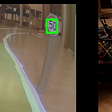## Random Forest Classifier- A Beginner’s Guide## Reinforcement learning assisted search ranking## An Introduction to Natural Language Processing## Solving Navigation using Deep Reinforcement Learning (Value-Based Methods)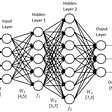## WTF is Convergence? — AWS DeepRacer## Federico Pichi

Ph.D. in Mathematical Analysis, Modelling and Applications, mathLab group, Mathematics Area, SISSA, International School for Advanced Studies

## Approaches to Semi-Supervised Image Classification: Virtual Adversarial Training, MixMatch## Loan Default Prediction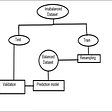## Experience with Group Project